# 欢迎关注WX公众号：【程序员管小亮】

【机器学习】《机器学习实战》读书笔记及代码 总目录

GitHub代码地址：

——————————————————————————————————————————————————————

## 本章内容

• Sigmoid函数和Logistic回归分类器
• 最优化理论初步
• 梯度下降最优化算法
• 数据中的缺失项处理

### 1、Logistic 回归Logistic回归的一般过程
(1) 收集数据：采用任意方法收集数据。
(2) 准备数据：由于需要进行距离计算，因此要求数据类型为数值型。另外，结构化数据格式则最佳。
(3) 分析数据：采用任意方法对数据进行分析。
(4) 训练算法：大部分时间将用于训练，训练的目的是为了找到最佳的分类回归系数。
(5) 测试算法：一旦训练步骤完成，分类将会很快。
(6) 使用算法：首先，我们需要输入一些数据，并将其转换成对应的结构化数值；接着，基于训练好的回归系数就可以对这些数值进行简单的回归计算，判定它们属于哪个类别；在这之后，我们就可以在输出的类别上做一些其他分析工作。

Logistic回归的因变量可以是二分类的，也可以是多分类的，但是实际中最为常用的就是二分类的Logistic回归。它利用的是Sigmoid函数阈值在[0,1]这个特性。Logistic回归进行分类的主要思想是：根据现有数据对分类边界线建立回归公式，以此进行分类。其实，Logistic本质上是一个基于条件概率的判别模型(Discriminative Model)。

Logistic回归

### 2、基于Logistic 回归和Sigmoid 函数的分类### 3、梯度上升算法

Sigmoid函数的输入记为z，由下面公式得出：### 4、基于最优化方法的最佳回归系数确定

#### 1）查看数据的分布情况import matplotlib.pyplot as plt
import numpy as np

"""
Parameters:
无
Returns:
dataMat - 数据列表
labelMat - 标签列表
"""
# 函数说明:加载数据
dataMat = []                                                        #创建数据列表
labelMat = []                                                       #创建标签列表
fr = open('testSet.txt')                                            #打开文件
lineArr = line.strip().split()                                  #去回车，放入列表
dataMat.append([1.0, float(lineArr), float(lineArr)])     #添加数据
labelMat.append(int(lineArr))                                #添加标签
fr.close()                                                          #关闭文件
return dataMat, labelMat                                            #返回

# 函数说明:绘制数据集
def plotDataSet():
dataArr = np.array(dataMat)                                         #转换成numpy的array数组
n = np.shape(dataMat)                                            #数据个数
xcord1 = []; ycord1 = []                                            #正样本
xcord2 = []; ycord2 = []                                            #负样本
for i in range(n):                                                  #根据数据集标签进行分类
if int(labelMat[i]) == 1:
xcord1.append(dataArr[i,1]); ycord1.append(dataArr[i,2])    #1为正样本
else:
xcord2.append(dataArr[i,1]); ycord2.append(dataArr[i,2])    #0为负样本
fig = plt.figure()
ax.scatter(xcord1, ycord1, s = 20, c = 'red', marker = 's',alpha=.5)#绘制正样本
ax.scatter(xcord2, ycord2, s = 20, c = 'green',alpha=.5)            #绘制负样本
plt.title('DataSet')                                                #绘制title
plt.xlabel('x'); plt.ylabel('y')                                    #绘制label
plt.show()                                                          #显示

if __name__ == '__main__':
plotDataSet()#### 2）训练算法：使用梯度上升找到最佳参数

每个回归系数初始化为1

计算整个数据集的梯度
返回回归系数


import numpy as np

'''
Parameters:
无
Returns:
dataMat - 数据列表
labelMat - 标签列表
'''
# 函数说明:加载数据
dataMat = []                                                    #创建数据列表
labelMat = []                                                   #创建标签列表
fr = open('testSet.txt')                                        #打开文件
lineArr = line.strip().split()                              #去回车，放入列表
dataMat.append([1.0, float(lineArr), float(lineArr)]) #添加数据
labelMat.append(int(lineArr))                            #添加标签
fr.close()                                                      #关闭文件
return dataMat, labelMat                                        #返回

'''
Parameters:
inX - 数据
Returns:
sigmoid函数
'''
# 函数说明:sigmoid函数
def sigmoid(inX):
return 1.0 / (1 + np.exp(-inX))

'''
Parameters:
dataMatIn - 数据集
classLabels - 数据标签
Returns:
'''
# 函数说明:梯度上升算法
dataMatrix = np.mat(dataMatIn)                                  #转换成numpy的mat
labelMat = np.mat(classLabels).transpose()                      #转换成numpy的mat,并进行转置
m, n = np.shape(dataMatrix)                                     #返回dataMatrix的大小。m为行数,n为列数。
alpha = 0.001                                                   #移动步长,也就是学习速率,控制更新的幅度。
maxCycles = 500                                                 #最大迭代次数
weights = np.ones((n,1))
for k in range(maxCycles):
h = sigmoid(dataMatrix * weights)                           #梯度上升矢量化公式
error = labelMat - h
weights = weights + alpha * dataMatrix.transpose() * error
return weights.getA()                                           #将矩阵转换为数组，返回权重数组

if __name__ == '__main__':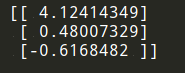#### 3）分析数据：画出决策边界

import matplotlib.pyplot as plt
import numpy as np

'''
Parameters:
无
Returns:
dataMat - 数据列表
labelMat - 标签列表
'''
# 函数说明:加载数据
dataMat = []                                                    #创建数据列表
labelMat = []                                                   #创建标签列表
fr = open('testSet.txt')                                        #打开文件
lineArr = line.strip().split()                              #去回车，放入列表
dataMat.append([1.0, float(lineArr), float(lineArr)]) #添加数据
labelMat.append(int(lineArr))                            #添加标签
fr.close()                                                      #关闭文件
return dataMat, labelMat                                        #返回

'''
Parameters:
inX - 数据
Returns:
sigmoid函数
'''
# 函数说明:sigmoid函数
def sigmoid(inX):
return 1.0 / (1 + np.exp(-inX))

'''
Parameters:
dataMatIn - 数据集
classLabels - 数据标签
Returns:
weights.getA() - 求得的权重数组(最优参数)
'''
# 函数说明:梯度上升算法
dataMatrix = np.mat(dataMatIn)                                  #转换成numpy的mat
labelMat = np.mat(classLabels).transpose()                      #转换成numpy的mat,并进行转置
m, n = np.shape(dataMatrix)                                     #返回dataMatrix的大小。m为行数,n为列数。
alpha = 0.001                                                   #移动步长,也就是学习速率,控制更新的幅度。
maxCycles = 500                                                 #最大迭代次数
weights = np.ones((n,1))
for k in range(maxCycles):
h = sigmoid(dataMatrix * weights)                           #梯度上升矢量化公式
error = labelMat - h
weights = weights + alpha * dataMatrix.transpose() * error
return weights.getA()                                           #将矩阵转换为数组，返回权重数组

'''
Parameters:
weights - 权重参数数组
Returns:
无
'''
# 函数说明:绘制数据集
def plotBestFit(weights):
dataArr = np.array(dataMat)                                         #转换成numpy的array数组
n = np.shape(dataMat)                                            #数据个数
xcord1 = []; ycord1 = []                                            #正样本
xcord2 = []; ycord2 = []                                            #负样本
for i in range(n):                                                  #根据数据集标签进行分类
if int(labelMat[i]) == 1:
xcord1.append(dataArr[i,1]); ycord1.append(dataArr[i,2])    #1为正样本
else:
xcord2.append(dataArr[i,1]); ycord2.append(dataArr[i,2])    #0为负样本
fig = plt.figure()
ax.scatter(xcord1, ycord1, s = 20, c = 'red', marker = 's',alpha=.5)#绘制正样本
ax.scatter(xcord2, ycord2, s = 20, c = 'green',alpha=.5)            #绘制负样本
x = np.arange(-3.0, 3.0, 0.1)
y = (-weights - weights * x) / weights
ax.plot(x, y)
plt.title('BestFit')                                                #绘制title
plt.xlabel('X1'); plt.ylabel('X2')                                  #绘制label
plt.show()

if __name__ == '__main__':
plotBestFit(weights)#### 4）训练算法：随机梯度上升

所有回归系数初始化为1

计算该样本的梯度



from matplotlib.font_manager import FontProperties
import matplotlib.pyplot as plt
import numpy as np
import random

'''
Parameters:
无
Returns:
dataMat - 数据列表
labelMat - 标签列表
'''
# 函数说明:加载数据
dataMat = []                                                    #创建数据列表
labelMat = []                                                   #创建标签列表
fr = open('testSet.txt')                                        #打开文件
lineArr = line.strip().split()                              #去回车，放入列表
dataMat.append([1.0, float(lineArr), float(lineArr)]) #添加数据
labelMat.append(int(lineArr))                            #添加标签
fr.close()                                                      #关闭文件
return dataMat, labelMat                                        #返回

'''
Parameters:
inX - 数据
Returns:
sigmoid函数
'''
# 函数说明:sigmoid函数
def sigmoid(inX):
return 1.0 / (1 + np.exp(-inX))

'''
Parameters:
weights - 权重参数数组
Returns:
无
'''
# 函数说明:绘制数据集
def plotBestFit(weights):
dataArr = np.array(dataMat)                                         #转换成numpy的array数组
n = np.shape(dataMat)                                            #数据个数
xcord1 = []; ycord1 = []                                            #正样本
xcord2 = []; ycord2 = []                                            #负样本
for i in range(n):                                                  #根据数据集标签进行分类
if int(labelMat[i]) == 1:
xcord1.append(dataArr[i,1]); ycord1.append(dataArr[i,2])    #1为正样本
else:
xcord2.append(dataArr[i,1]); ycord2.append(dataArr[i,2])    #0为负样本
fig = plt.figure()
ax.scatter(xcord1, ycord1, s = 20, c = 'red', marker = 's',alpha=.5)#绘制正样本
ax.scatter(xcord2, ycord2, s = 20, c = 'green',alpha=.5)            #绘制负样本
x = np.arange(-3.0, 3.0, 0.1)
y = (-weights - weights * x) / weights
ax.plot(x, y)
plt.title('BestFit')                                                #绘制title
plt.xlabel('X1'); plt.ylabel('X2')                                  #绘制label
plt.show()

'''
Parameters:
dataMatrix - 数据数组
classLabels - 数据标签
numIter - 迭代次数
Returns:
weights - 求得的回归系数数组(最优参数)
'''
# 函数说明:改进的随机梯度上升算法
m,n = np.shape(dataMatrix)                                       #返回dataMatrix的大小。m为行数,n为列数。
weights = np.ones(n)                                             #参数初始化
for j in range(numIter):
dataIndex = list(range(m))
for i in range(m):
alpha = 4/(1.0+j+i)+0.01                                 #降低alpha的大小，每次减小1/(j+i)。
randIndex = int(random.uniform(0,len(dataIndex)))        #随机选取样本
h = sigmoid(sum(dataMatrix[randIndex]*weights))          #选择随机选取的一个样本，计算h
error = classLabels[randIndex] - h                       #计算误差
weights = weights + alpha * error * dataMatrix[randIndex]#更新回归系数
del(dataIndex[randIndex])                                #删除已经使用的样本
return weights                                                   #返回

if __name__ == '__main__':
plotBestFit(weights)#### 5）回归系数与迭代次数的关系

from matplotlib.font_manager import FontProperties
import matplotlib.pyplot as plt
import numpy as np
import random

'''
Parameters:
无
Returns:
dataMat - 数据列表
labelMat - 标签列表
'''
# 函数说明:加载数据
dataMat = []                                                    #创建数据列表
labelMat = []                                                   #创建标签列表
fr = open('testSet.txt')                                        #打开文件
lineArr = line.strip().split()                              #去回车，放入列表
dataMat.append([1.0, float(lineArr), float(lineArr)]) #添加数据
labelMat.append(int(lineArr))                            #添加标签
fr.close()                                                      #关闭文件
return dataMat, labelMat                                        #返回

'''
Parameters:
inX - 数据
Returns:
sigmoid函数
'''
# 函数说明:sigmoid函数
def sigmoid(inX):
return 1.0 / (1 + np.exp(-inX))

'''
Parameters:
dataMatIn - 数据集
classLabels - 数据标签
Returns:
'''
# 函数说明:梯度上升算法
dataMatrix = np.mat(dataMatIn)                                  #转换成numpy的mat
labelMat = np.mat(classLabels).transpose()                      #转换成numpy的mat,并进行转置
m, n = np.shape(dataMatrix)                                     #返回dataMatrix的大小。m为行数,n为列数。
alpha = 0.001                                                   #移动步长,也就是学习速率,控制更新的幅度。
maxCycles = 500                                                 #最大迭代次数
weights = np.ones((n,1))
for k in range(maxCycles):
h = sigmoid(dataMatrix * weights)                           #梯度上升矢量化公式
error = labelMat - h
weights = weights + alpha * dataMatrix.transpose() * error
return weights.getA()                                           #将矩阵转换为数组，返回权重数组

'''
Parameters:
dataMatrix - 数据数组
classLabels - 数据标签
numIter - 迭代次数
Returns:
weights - 求得的回归系数数组(最优参数)
'''
# 函数说明:改进的随机梯度上升算法
m,n = np.shape(dataMatrix)                                       #返回dataMatrix的大小。m为行数,n为列数。
weights = np.ones(n)                                             #参数初始化
for j in range(numIter):
dataIndex = list(range(m))
for i in range(m):
alpha = 4/(1.0+j+i)+0.01                                 #降低alpha的大小，每次减小1/(j+i)。
randIndex = int(random.uniform(0,len(dataIndex)))        #随机选取样本
h = sigmoid(sum(dataMatrix[randIndex]*weights))          #选择随机选取的一个样本，计算h
error = classLabels[randIndex] - h                       #计算误差
weights = weights + alpha * error * dataMatrix[randIndex]#更新回归系数
del(dataIndex[randIndex])                                #删除已经使用的样本
return weights                                                   #返回

'''
Parameters:
weights_array1 - 回归系数数组1
weights_array2 - 回归系数数组2
Returns:
无
'''
# 函数说明:绘制回归系数与迭代次数的关系
def plotWeights(weights_array1,weights_array2):
#设置汉字格式
font = FontProperties(fname=r"c:\windows\fonts\simsun.ttc", size=14)
#将fig画布分隔成1行1列,不共享x轴和y轴,fig画布的大小为(13,8)
#当nrow=3,nclos=2时,代表fig画布被分为六个区域,axs表示第一行第一列
fig, axs = plt.subplots(nrows=3, ncols=2,sharex=False, sharey=False, figsize=(20,10))
x1 = np.arange(0, len(weights_array1), 1)
#绘制w0与迭代次数的关系
axs.plot(x1,weights_array1[:,0])
axs0_title_text = axs.set_title(u'梯度上升算法：回归系数与迭代次数关系',FontProperties=font)
axs0_ylabel_text = axs.set_ylabel(u'W0',FontProperties=font)
plt.setp(axs0_title_text, size=20, weight='bold', color='black')
plt.setp(axs0_ylabel_text, size=20, weight='bold', color='black')
#绘制w1与迭代次数的关系
axs.plot(x1,weights_array1[:,1])
axs1_ylabel_text = axs.set_ylabel(u'W1',FontProperties=font)
plt.setp(axs1_ylabel_text, size=20, weight='bold', color='black')
#绘制w2与迭代次数的关系
axs.plot(x1,weights_array1[:,2])
axs2_xlabel_text = axs.set_xlabel(u'迭代次数',FontProperties=font)
axs2_ylabel_text = axs.set_ylabel(u'W1',FontProperties=font)
plt.setp(axs2_xlabel_text, size=20, weight='bold', color='black')
plt.setp(axs2_ylabel_text, size=20, weight='bold', color='black')

x2 = np.arange(0, len(weights_array2), 1)
#绘制w0与迭代次数的关系
axs.plot(x2,weights_array2[:,0])
axs0_title_text = axs.set_title(u'改进的随机梯度上升算法：回归系数与迭代次数关系',FontProperties=font)
axs0_ylabel_text = axs.set_ylabel(u'W0',FontProperties=font)
plt.setp(axs0_title_text, size=20, weight='bold', color='black')
plt.setp(axs0_ylabel_text, size=20, weight='bold', color='black')
#绘制w1与迭代次数的关系
axs.plot(x2,weights_array2[:,1])
axs1_ylabel_text = axs.set_ylabel(u'W1',FontProperties=font)
plt.setp(axs1_ylabel_text, size=20, weight='bold', color='black')
#绘制w2与迭代次数的关系
axs.plot(x2,weights_array2[:,2])
axs2_xlabel_text = axs.set_xlabel(u'迭代次数',FontProperties=font)
axs2_ylabel_text = axs.set_ylabel(u'W1',FontProperties=font)
plt.setp(axs2_xlabel_text, size=20, weight='bold', color='black')
plt.setp(axs2_ylabel_text, size=20, weight='bold', color='black')

plt.show()

if __name__ == '__main__':

plotWeights(weights_array1, weights_array2)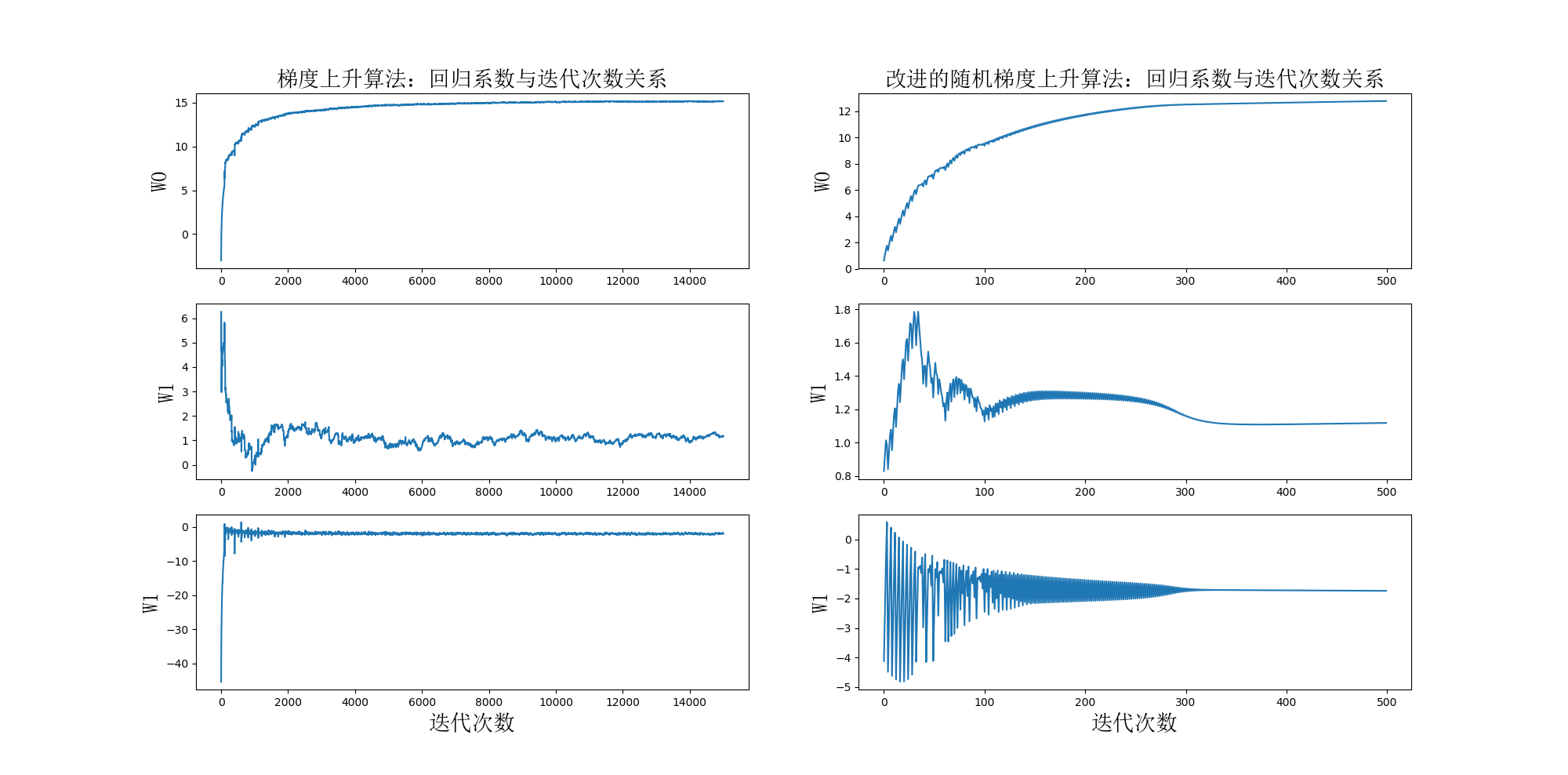### 5、示例：从疝气病症预测病马的死亡率

(1) 收集数据：给定数据文件。
(2) 准备数据：用Python解析文本文件并填充缺失值。
(3) 分析数据：可视化并观察数据。
(4) 训练算法：使用优化算法，找到最佳的系数。
(5) 测试算法：为了量化回归的效果，需要观察错误率。根据错误率决定是否回退到训练阶段，通过改变迭代的次数和步长等参数来得到更好的回归系数。
(6) 使用算法：实现一个简单的命令行程序来收集马的症状并输出预测结果并非难事。

#### 1）准备数据：处理数据中的缺失值

• 使用可用特征的均值来填补缺失值；
• 使用特殊值来填补缺失值，如 -1；
• 忽略有缺失值的样本；
• 使用相似样本的均值添补缺失值；
• 使用另外的机器学习算法预测缺失值。

• 如果测试集中一条数据的特征值已经确实，那么我们选择实数0来替换所有缺失值，因为本文使用Logistic回归。因此这样做不会影响回归系数的值。sigmoid(0)=0.5，即它对结果的预测不具有任何倾向性。
• 如果测试集中一条数据的类别标签已经缺失，那么我们将该类别数据丢弃，因为类别标签与特征不同，很难确定采用某个合适的值来替换。

#### 2）测试算法：用Logistic 回归进行分类

import numpy as np
import random

'''
Parameters:
inX - 数据
Returns:
sigmoid函数
'''
# 函数说明:sigmoid函数
def sigmoid(inX):
return 1.0 / (1 + np.exp(-inX))

'''
Parameters:
dataMatrix - 数据数组
classLabels - 数据标签
numIter - 迭代次数
Returns:
weights - 求得的回归系数数组(最优参数)
'''
# 函数说明:改进的随机梯度上升算法
m,n = np.shape(dataMatrix)                                         #返回dataMatrix的大小。m为行数,n为列数。
weights = np.ones(n)                                               #参数初始化
#存储每次更新的回归系数
for j in range(numIter):
dataIndex = list(range(m))
for i in range(m):
alpha = 4/(1.0+j+i)+0.01                                  #降低alpha的大小，每次减小1/(j+i)。
randIndex = int(random.uniform(0,len(dataIndex)))         #随机选取样本
h = sigmoid(sum(dataMatrix[randIndex]*weights))           #选择随机选取的一个样本，计算h
error = classLabels[randIndex] - h                        #计算误差
weights = weights + alpha * error * dataMatrix[randIndex] #更新回归系数
del(dataIndex[randIndex])                                 #删除已经使用的样本
return weights                                                    #返回

# 函数说明:使用Python写的Logistic分类器做预测
def colicTest():
frTrain = open('horseColicTraining.txt')                                          #打开训练集
frTest = open('horseColicTest.txt')                                               #打开测试集
trainingSet = []; trainingLabels = []
currLine = line.strip().split('\t')
lineArr = []
for i in range(len(currLine)-1):
lineArr.append(float(currLine[i]))
trainingSet.append(lineArr)
trainingLabels.append(float(currLine[-1]))
trainWeights = stocGradAscent1(np.array(trainingSet), trainingLabels, 500)        #使用改进的随即上升梯度训练
errorCount = 0; numTestVec = 0.0
numTestVec += 1.0
currLine = line.strip().split('\t')
lineArr =[]
for i in range(len(currLine)-1):
lineArr.append(float(currLine[i]))
if int(classifyVector(np.array(lineArr), trainWeights))!= int(currLine[-1]):
errorCount += 1
errorRate = (float(errorCount)/numTestVec) * 100                                 #错误率计算
print("测试集错误率为: %.2f%%" % errorRate)

'''
Parameters:
inX - 特征向量
weights - 回归系数
Returns:
分类结果
'''
# 函数说明:分类函数
def classifyVector(inX, weights):
prob = sigmoid(sum(inX*weights))
if prob > 0.5: return 1.0
else: return 0.0

if __name__ == '__main__':
colicTest()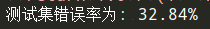import numpy as np
import random

'''
Parameters:
inX - 数据
Returns:
sigmoid函数
'''
# 函数说明:sigmoid函数
def sigmoid(inX):
return 1.0 / (1 + np.exp(-inX))

'''
Parameters:
dataMatIn - 数据集
classLabels - 数据标签
Returns:
weights.getA() - 求得的权重数组(最优参数)
'''
# 函数说明:梯度上升算法
dataMatrix = np.mat(dataMatIn)                                 #转换成numpy的mat
labelMat = np.mat(classLabels).transpose()                     #转换成numpy的mat,并进行转置
m, n = np.shape(dataMatrix)                                    #返回dataMatrix的大小。m为行数,n为列数。
alpha = 0.01                                                   #移动步长,也就是学习速率,控制更新的幅度。
maxCycles = 500                                                #最大迭代次数
weights = np.ones((n,1))
for k in range(maxCycles):
h = sigmoid(dataMatrix * weights)                          #梯度上升矢量化公式
error = labelMat - h
weights = weights + alpha * dataMatrix.transpose() * error
return weights.getA()                                          #将矩阵转换为数组，并返回

# 函数说明:使用Python写的Logistic分类器做预测
def colicTest():
frTrain = open('horseColicTraining.txt')                                          #打开训练集
frTest = open('horseColicTest.txt')                                               #打开测试集
trainingSet = []; trainingLabels = []
currLine = line.strip().split('\t')
lineArr = []
for i in range(len(currLine)-1):
lineArr.append(float(currLine[i]))
trainingSet.append(lineArr)
trainingLabels.append(float(currLine[-1]))
trainWeights = stocGradAscent1(np.array(trainingSet), trainingLabels, 500)        #使用改进的随即上升梯度训练
errorCount = 0; numTestVec = 0.0
numTestVec += 1.0
currLine = line.strip().split('\t')
lineArr =[]
for i in range(len(currLine)-1):
lineArr.append(float(currLine[i]))
if int(classifyVector(np.array(lineArr), trainWeights))!= int(currLine[-1]):
errorCount += 1
errorRate = (float(errorCount)/numTestVec) * 100                                 #错误率计算
print("测试集错误率为: %.2f%%" % errorRate)

'''
Parameters:
inX - 特征向量
weights - 回归系数
Returns:
分类结果
'''
# 函数说明:分类函数
def classifyVector(inX, weights):
prob = sigmoid(sum(inX*weights))
if prob > 0.5: return 1.0
else: return 0.0

if __name__ == '__main__':
colicTest()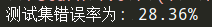• 当数据集较小时，使用梯度上升算法
• 当数据集较大时，使用改进的随机梯度上升算法

• 数据较小的时候，我们使用liblinear
• 数据较大时，我们使用sag和saga

### 6、Sklearn构建Logistic回归分类器

from sklearn.linear_model import LogisticRegression

# 函数说明:使用Sklearn构建Logistic回归分类器
def colicSklearn():
frTrain = open('horseColicTraining.txt')              #打开训练集
frTest = open('horseColicTest.txt')                   #打开测试集
trainingSet = []; trainingLabels = []
testSet = []; testLabels = []
currLine = line.strip().split('\t')
lineArr = []
for i in range(len(currLine)-1):
lineArr.append(float(currLine[i]))
trainingSet.append(lineArr)
trainingLabels.append(float(currLine[-1]))
currLine = line.strip().split('\t')
lineArr =[]
for i in range(len(currLine)-1):
lineArr.append(float(currLine[i]))
testSet.append(lineArr)
testLabels.append(float(currLine[-1]))
classifier = LogisticRegression(solver='liblinear',max_iter=10).fit(trainingSet, trainingLabels)
test_accurcy = classifier.score(testSet, testLabels) * 100
print('正确率:%f%%' % test_accurcy)

if __name__ == '__main__':
colicSklearn()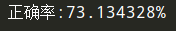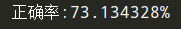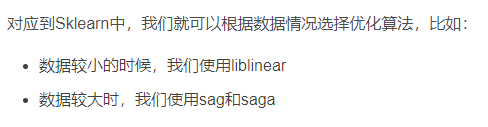### 7、sklearn.linear_model.LogisticRegression

sklearn.linear_model.LogisticRegression是一个很好的模型，决策树算法就是通过它实现的，详细的看这个博客——sklearn.linear_model.LogisticRegression()函数解析（最清晰的解释）

### 8、总结

Logistic回归的目的是寻找一个非线性函数Sigmoid的最佳拟合参数，求解过程可以由最优化算法来完成。在最优化算法中，最常用的就是 梯度上升算法，而 梯度上升算法 又可以简化为 随机梯度上升算法

### 参考文章

11-2310-224179
08-303万+
11-171万+
12-03
08-195019